# Excess coefficient

coefficient of excess, excess

A scalar characteristic of the pointedness of the graph of the probability density of a unimodal distribution. It is used as a certain measure of the deviation of the distribution in question from the normal one. The excessis defined by the formula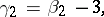whereis the second Pearson coefficient (cf. Pearson distribution), and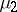andare the second and fourth central moments of the probability distribution. In terms of the second- and fourth-order semi-invariants (cumulants)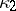and, the excess has the form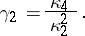If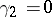, then one says that the density of the probability distribution has normal excess, because for a normal distribution the excess is. When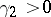, one says that the probability distribution has positive excess, which corresponds, as a rule, to the fact that the graph of the density of the relevant distribution in a neighbourhood of the mode has a more pointed and higher vertex then a normal curve. When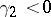, one talks of a negative excess of the density, and then the probability density in a neighbourhood of the mode has a lower and flatter vertex than the density of a normal law.

Ifare independent random variables subject to one and same continuous probability law, then the statistic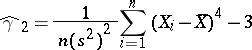is called the sample excess, whereThe sample excessis used as a statistical point estimator of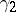when the distribution law of the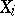is not known. In the case of a normal distribution of the random variables, the sample excess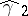is asymptotically normally distributed, as, with parametersandThis is the reason why, when the observed value ofdiffers substantially from, one must assume that the distribution of theis not normal. This is used in practice to verify the hypothesis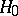: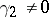, which is equivalent to the fact that the distribution of thedeviates from the normal distribution.